# 【DSE Physics Formula Sheet】物理公式表 (中文+English)## DSE 物理公式表中文版 (by topic 整理)

###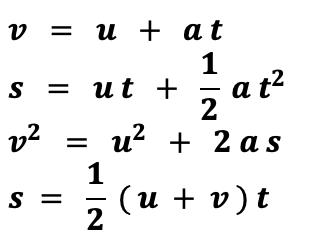where s = 位移 (+/—ive) (單位: m)

where u, v = 速度 (+/—ive) (單位: m s—1)

where a = 加速度 (+/—ive) (單位: m s—1)

Formulas A1-A5

### 溫度 & 物態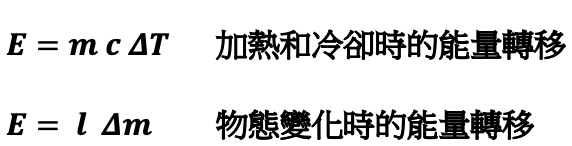where E = 能量 (單位: J)

where m = 質量 (單位: kg)

where c = 比熱容量 (單位: J kg—1 K—1)

where l = 潛熱 (單位:  J kg—1)

### 理想氣體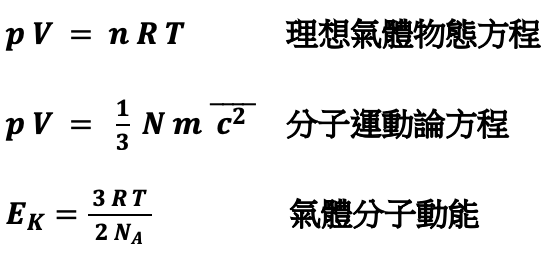where p = 壓強 (單位: Pa)

where V = 體積 (單位: m3)

where R = 8.31 (單位: J mol—1 K—1)

where T = 溫度 (單位: K)

where kB = 1.38 × 10-23  (單位: J K—1) ;

where EK = 動能 (單位: J)

where NA = 6.02 x 1023 (單位: mol—1)

Formulas B1-B7

### 動量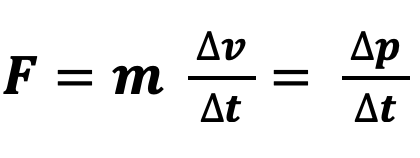where F = 力 (單位: N)

where m = 質量 (單位: kg)

### 轉矩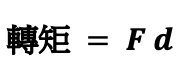where F = 力 (單位: N)

### 能量 & 功率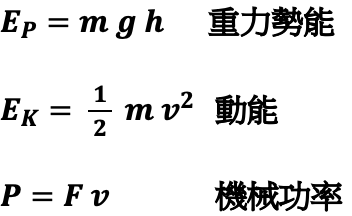where Ep = 重力勢能 (單位: J)

where m = 質量 (單位: kg)

where g = 9.81 (單位: m s—2)

where Ek = 動能 (單位: J)

where P = 機械功率 (單位: W)

where F = 施加力 (單位: N)

### 圓週運動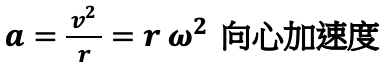where a = 向心加速度 (單位: m s—2)

where ω = 角速度 (單位: rad s—1)

where f = 頻率 (單位: Hz)

where F = 向心力 (單位:  N)

###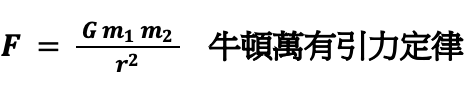where  F = 量值引力 (單位: N)

where G = 6.67 × 10-11 (單位: N m2 kg—2)

where m1, m2 = 質量 (單位: kg)

where r = 距離 (單位: m)

Formulas C1-C3

### 雙狹縫 & 衍射光柵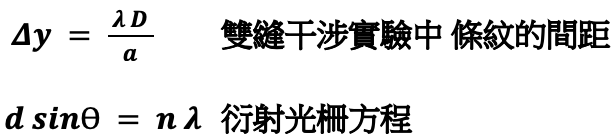where  Δy = 條紋之間的距離 (單位: m)

where λ = 波長 (單位: m)

where D = 雙狹縫與條紋之間的距離 (單位: m)

where a = 雙狹縫之間的距離 (單位: m)

where d = 衍射光柵之間的距離 (單位: m)

where n = 0, 1, 2, 3

### 透鏡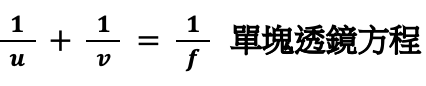where u = 物距 (單位: m)

where v = 像距 (單位: m)

where f = 焦距  (單位: m)

Formulas D1-D13

### 電場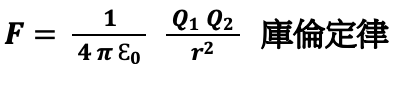where F = 靜電力 (單位: N)

where Ɛ0 = 8.85 × 10-12 (單位: C2 N—1 m—2)

where Q1, Q2  = 電荷 (單位: C)

where r = 距離 (單位: m)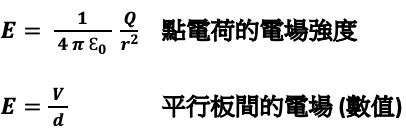where E = 電場強度 (單位: N C—1  or  V m—1 )

where Q = 電荷 (單位: C)

where V = 電勢差 (單位: V)

where d = 平行板間的距離 (單位: m)

### 電阻率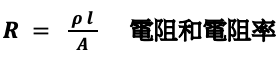where R = 電阻 (單位: Ω)

where ρ = 電阻率 (單位: Ωm)

where l = 長度 (單位: m)

where A = 橫截面面積  (單位: m2)

###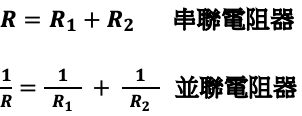where R = 等效電阻 (單位: Ω)

### 電路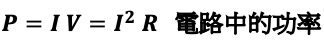where P = 功率 (單位: W)

where I = 電流 (單位: A)

where V = 電勢差 (單位: V)

where R = 電阻 (單位: Ω)

### 磁場對運動電荷的作用力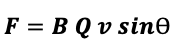where F = 力 (單位: N)

where B = 磁場強度 (單位: T)

where Q = 電荷 (單位: C)

### 磁場對載流導體的作用力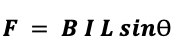where F = 力 (單位: N)

where B = 磁場強度 (單位: T)

where I = 電流 (單位: A)

where L = 長度 (單位: m)

### 長直導線所產生的磁場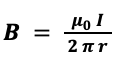where B = 磁場強度 (單位: T)

where μ0 = 4π × 10-7 (單位: H m—1)

where I = 電流 (單位: A)

where r = 距離 (單位: m)

### 螺線管中的磁場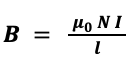where B = 磁場強度 (單位: T)

where μ0 = 4π × 10-7 (單位: H m—1)

where I = 電流 (單位: A)

where l = 螺線管長度 (單位: m)

### 感生電動勢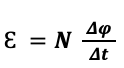where ε = 感應電動勢

where Δφ = 磁通量的改變 (單位: Wb)

where Δt = 時間間隔 (單位: s)

where φ = 磁通量(單位: Wb)

### 變壓器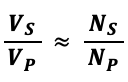where VS = 副線圈電勢差(單位: V)

where VP = 原線圈電勢差(單位: V)

where NS = 副線圈線圈數量

where NP = 原線圈線圈數量

Formulas E1-E4

###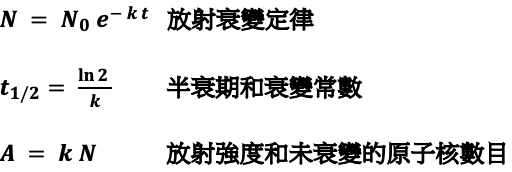where N = 剩餘的不衰變放射性核

where k = 衰變/放射常數 (單位: s—1)

where t = 時間間隔 (單位: s)

where t1/2 = 半衰期 (單位: s)

###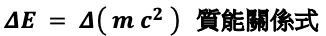where E = 能量 (單位: J or eV)

where m = 質量 (單位: kg or eV/c2 )

where c = 3 × 108 (單位: m s—1)

## DSE Physics Formula Sheet English Version (by topic)

###where s = displacement (+/—ive) (units: m)

where u, v = initial, final velocity (+/—ive) (units: m s—1)

where a = acceleration (+/—ive) (units: m s—1)

where t = time (≥ 0)  (units: s)

Formulas A1-A5

### Temperature & State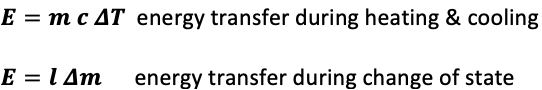where E = energy (units: J)

where m = mass (units: kg)

where c = specific heat capacity (units: J kg—1 K—1)

where ΔT = change in temperature (units: K)

where l = latent heat (units:  J kg—1)

### Ideal Gas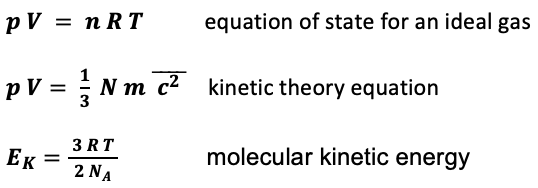where p = pressure (units: Pa

where V = volume (units: m3)

where n = number of moles of gas

where R = 8.31 (units: J mol—1 K—1)

where T = temperature (units: K)

where N = number of gas molecules

where kB = 1.38 × 10-23 (units: J K—1)

where EK = KE (units: J)

where NA = 6.02 x 1023 (units: mol—1)

Formulas B1-B7

### Force & Momentumwhere F = force (units: N)

where m = mass (units: kg)

### Moments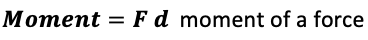where F = force (units: N)

### Energy & Power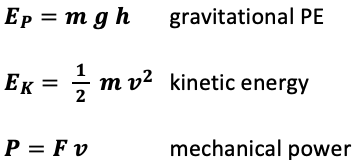where EP = gravitational PE (units: J)

where m = mass (units: kg )

where g = 9.81 (units: m s—2)

where EK = KE (units: J)

where P = mechanical power (units: W)

where F = applied force (units: N)

###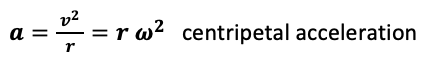where a = centripetal acceleration (units: m s—2)

where v = speed (units: m s—1)

Where r = radius (units: m)

Where ω = angular velocity (units: rad s—1)

where f = frequency (units: Hz)

where F = centripetal force (units:  N)

###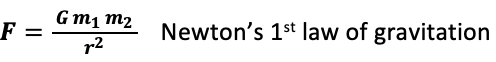where F = gravitational force (units: N)

where G = 6.67 × 10-11 (units: N m2 kg—2

where m1, m2 = mass (units: kg)

where r = separation (units: m)

Formulas C1-C3

###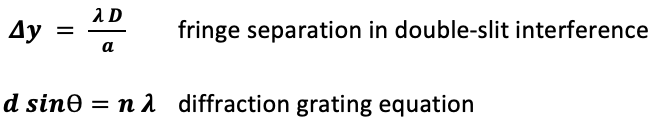where Δy = separation between fringes (units: m)

where λ = wavelength (units: m)

where D = separation between the double-slit & the screen (units: m)

where a = separation between the slits  (units: m

where d = separation between each grating (units: m)

where n = order = 0, 1, 2, 3

### Lenses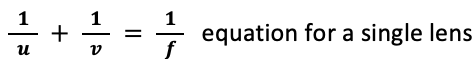where u = object distance (units: m)

where v = image distance (units: m)

where f = focal length (units: m)

Formulas D1-D13

### Electric Fields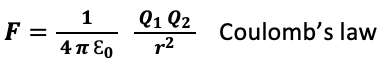where F = electrostatic force (units: N)

where Ɛ0 = 8.85 × 10-12 (units: C2 N—1 m—2)

where Q1, Q2 = electric charge (units: C)

where r = separation (units: m)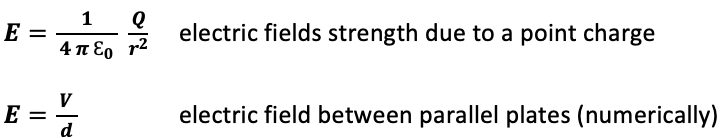where E = electric field strength (units: N C—1 or  V m—1)

where Q = charge (units: C)

where V = potential difference (units: V)

where d = separation (units: m)

### Resistivitywhere R = resistance (units: Ω)

where ρ = resistivity (units: Ωm)

where l = length (units: m)

where A = cross sectional area (units: m2)

### Resistors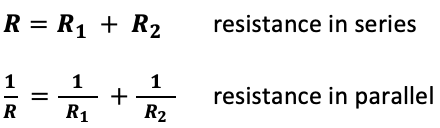where R = equivalent resistance (units: Ω

### Electric Circuits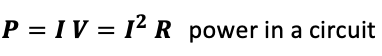where P = power (units: W)

where I = current (units: A)

where V = potential difference (units: V)

where R = resistance (units: Ω)

### Force on a Moving Charge in a Magnetic Field / B-field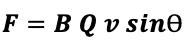where F = magnetic force (units: N)

where B = magnetic field strength (units: T)

where Q = charge (units: C)

### Force on a Current-carrying Conductor in a Magnetic Field/ B-field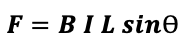where F = magnetic force (units: N)

where B = magnetic field strength (units: T)

where I = current (units: A)

where L = length (units: m)

###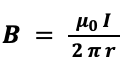where B = magnetic field strength  (units: T)

where μ0 = 4π × 10-7 (units: H m—1)

where I = current (units: A)

where r = distance (units: m)

### Magnetic Field/ B-field due to a Long Solenoid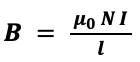where B = magnetic field strength (units: T)

where μ0 = 4π × 10-7 (units: H m—1)

where I = current (units: A)

where l = total length of the solenoid (units: m)

### Induced e.m.f.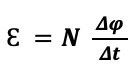where ε = induced e.m.f

where Δφ = change in magnetic flux (units: Wb)

where Δt = time interval (units: s)

where φ = magnetic flux (units: Wb)

### Transformers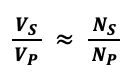where VS = potential difference across the Secondary coil (units: V)

where VP = potential difference across the Primary coil (units: V)

where NS = number of turns in the Secondary coil

where NP = number of turns/ windings in the Primary coil

Formulas E1-E4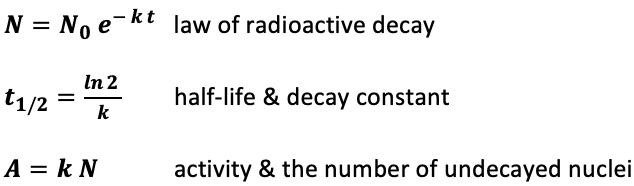where N = number of undecayed radioactive nuclei

where k = decay constant (units: s—1)

where t = time (units: s)

where t1/2 = half-life (units: s)

where A = activity (units: Bq)

### Energy-mass Equivalence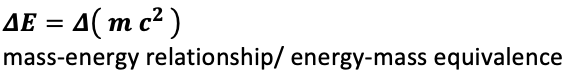where E = energy (units: J  or  eV)

where m = mass (units: kg  or  eV/c2 )

where c = 3 × 108 (units: m s—1)

## DSE Physcis 公式表應用建議

「點解要溫呢張 Formula Sheet 啊？」

Physics 嘅 formula 最麻煩就係就算你用啱條公式，但擺錯 value 嘅話都係「係咁先」！

Lenses 呢課入面嘅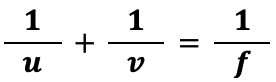（答案係 real image v > 0, virtual image v < 0, convex lens f > 0, concave lens f < 0）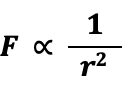「應該點溫呢張嘢阿？」AfterSchool

AfterSchool 是一個針對DSE而設的網上補習平台，課程涵蓋多個 DSE 科目，讓同學足不出戶就可以享有優質教學。註冊用戶人達 50,000 人，當中超過四分之一學生為應屆文憑試考生，是全港最大型網上補習平台。